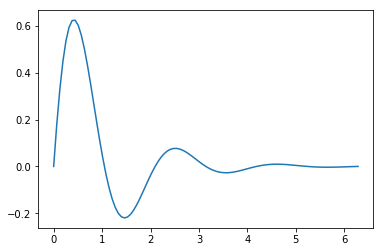Codex Vanitate archive

## Displaying Markdown and Latex in Jupyter output cells

Categories: Journal
Tags: ,
Published on: August 14, 2018

Just out of curiosity I started looking into how to get jupyter to display text and math formatted as markdown and latex in output cells. This got into my head when I was looking at symbolic integration and differentiation of functions and how to format the output in a more civilized manner. I don’t have the full solution to the question but formatting output as markdown turned out to be ridiculously easy since the heavy lifting had already been done.

### Displaying markdown and Latex in jupyter output cells¶

$$\phi$$

• And of course the new WordPress editor is helpfully mangling both the code highlighting and output. Trust me, it works. I’ll have to sort out how to get “Gutenberg” to do the right thing later.
• Thrashing around a bit now get’s the code highlighted but now the crayon tag is visible. Still no love for the actual Latex.
• Rolling back to the old editor and fixing these posts. I’ll deal with the gutenberg editor issues when I’m dragged kicking and screaming to it

## So far this morning

Categories: Journal, Projects
Tags:
Published on: August 11, 2018

So far this morning I’ve

• Gotten jupyter notebooks running natively on my workstation rather than using the linux subsystem as a workaround
• Learned how to integrate functions in python using scipy
• Got exporting of jupyter notebooks to html working properly
• Built a scale model of the terrain around my home town in Unity
• Gained three levels on my warlock

Now I’m going to put my pants on.

## Integrating Functions

Quick example on integrating functions with jupyter, matplotlib, numpy, and scipy

### Import the required libraries

For this example we’ll be using $$f(x) = e^{-x}sin(3x)$$ as our function.

### Set the range for your plot and integration

To plot $$f(x)$$ we get an array of linearly spaced values from numpy, pass that array and our function to the plot function, and show the plot.

Evalating the integral $$\int_{0}^{2\pi}e^{-x}sin(3x)$$ simply requires passing our function and the range of the intgral to scipy’s quad function which will return a tuple of the integral and the estimated error.Integral: 0.29943976718048754
Estimated error 5.05015300411582e-13

## Plugin testing

Categories: Journal
Tags: ,
\begin{align} J(\theta) &= \frac{1}{m}\sum_{i=1}^{m}Cost(h_\theta(x)^{(i)},y^{(i)}) \\ Cost(h_\theta(x),y) &= -log(h_\theta(x))& y=1 \\ Cost(h_\theta(x),y) &= -log(1-h_\theta(x))& y=0 \end{align}